Chemguide: Support for CIE A level Chemistry ``` ``` Learning outcome 7.1 Equilibria Chemical equilibria: reversible reactions, dynamic equilibrium ``` ``` ``` ``` Statement 7.1.7 This statement deals with calculating values for Kc and Kp from given data. Before you go on, you should find and read the statement in your copy of the syllabus. ``` ``` Obviously, before you can do this, you need to be confident about writing expressions for equilibrium constants as covered in statements 7.1.4 and 7.1.5. If you can't do that, go back and look at it again. If you are given the values for all of the concentrations or partial pressures, then this is simply a matter of slotting the numbers into the right equilibrium constant expression. But you also have to work out what the units are. Unfortunately, it is more likely that you won't be given all the numbers that you need, and will have to calculate one or more of them. This isn't actually very difficult, but there are so many different variations on this, that it is impossible to give them all on this page. My chemistry calculations book has no fewer than 14 pages looking at finding values for Kc and Kp including their units. I can't repeat this here. You will also find lots of examples in CIE's Chemistry Coursebook, together with end-of-chapter questions. Calculations are much better learnt from books than on the web. ``` ``` Statement 7.1.8 This statement expects you to be able to calculate the amounts of substances present at equilibrium if you are given the equilibrium constant. Before you go on, you should find and read the statement in your copy of the syllabus. My chemistry calculation book only has one example which looks like the ones that CIE have asked so far. The syllabus says (in 7.1.7) that you won't be given any question which needs you to solve quadratic equations, but even without having to do this, you need to be reasonably comfortable with simple algebra. We will look at two straightforward examples involving Kc. ``` ``` Example 1 The value of Kc for the formation of ethyl ethanoate from ethanoic acid and ethanol at 298 K is 4.0.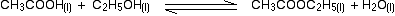If you mixed together 1.0 mol of each of ethanoic acid and ethanol at 298 K, calculate how many moles of each substance would be present in the equilibrium mixture. There is a standard way of setting out calculations of this kind which helps you to find your way through the calculation. Start by writing down the equilibrium equation with the number of moles of everything you start with written underneath it.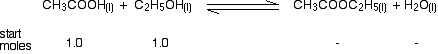Now write down how many moles of each substance there are at equilibrium. That's what we are trying to find at the end of the calculation, of course, so we can't literally do that at the moment. What we can do, however, is do it in terms of an unknown - x, for example. Suppose x moles of ethanoic acid have been used up at equilibrium. The number of moles of ethanoic acid left will be 1.0 - x. For every mole of ethanoic acid that is used up, the same number of moles of ethanol will have gone, because they react 1 to 1. There will be 1.0 - x moles of ethanol left as well. And for each mole of ethanoic acid that gets used up 1 mole of ethyl ethanoate and 1 mole of water are formed. So if x moles of ethanoic acid are used up, x moles of ethyl ethanoate and x moles of water will be formed. So the next line of the calculation looks like this: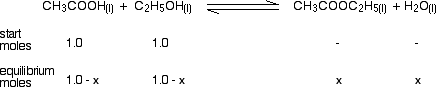The next problem is that equilibrium constant, Kc, needs values for concentration, not number of moles. If you knew the volume of the mixture, that would be simple to sort out, but in this example, you don't know it. At the time of writing, CIE have always told you the volume in their questions, even if in one case it was just "V". If you aren't told, just use V anyway. Your calculation so far now looks like this: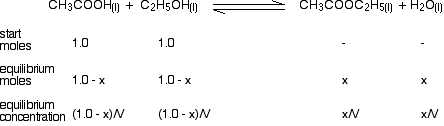You have now got all the necessary values to use in the equilibrium constant expression: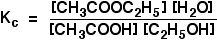If you put the concentration values into this expression, and remember that you were told the value of Kc was 4.0, you get the following expression: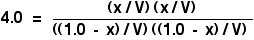When you sort this out, you will find that all the V terms cancel out, and you are left with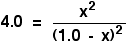Now for the clever bit! The obvious thing to do with this expression is to turn it into a quadratic equation of the form: ax2 + bx + c = 0 . . . and solve this using a formula. However, CIE don't want you do do this, and so there must be a simpler way. If you look at the right-hand side of the expression we have derived, you can see that it has perfect squares on both top and bottom. That means that you can simplify the equation by taking the square root of both sides. That gets rid of all the awkward square terms, and leaves you with a straightforward bit of algebra.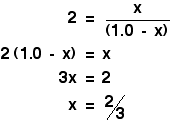That means that at equilibrium you have got: 2/3 of a mole of both the ethyl ethanoate and the water. (1 - 2/3) of a mole (= 1/3 of a mole) of both the ethanoic acid and ethanol. Warning!  We are making a hidden assumption in this which could produce a wrong answer. When you take the square root of 4, the answer could either be +2 or -2. We have assumed in this example that the answer was +2. What if it had been -2? If you work out the final bit of algebra using -2, you get the answer that x = 2 moles rather than x = 2/3 of a mole. How do you know which answer is right? It is essential when you do these calculations to ask yourself whether your answer is sensible. In this case, if you started with just 1 mole of ethanoic acid, could 2 moles of it get used up? Of course not! However, an answer of 2/3 of a mole used up is an entirely sensible result. If you choose the wrong root to work from, you will always get a silly answer. It might be obviously silly (for example, by being negative), or it may be less obvious until you think about it (for example, that you use up more than you started with). The positive root usually seems to give the right answer, so it makes sense to start with that one - but I wouldn't like to guarantee that that is always true. Example 2 The value of Kc for the formation of hydrogen iodide from hydrogen and iodine at a particular temperature is 64.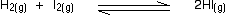If you mixed together 1 mol of each of hydrogen, iodine and hydrogen iodide at this temperature in a container of volume 25 dm3, calculate how many moles of each substance would be present in the equilibrium mixture. This is very similar to the last problem. You are given the volume this time, although it doesn't really matter, because it will cancel out again. There are two differences which you have to be careful with. One is that you are starting with some moles of everything, rather than just the substances on the left-hand side of the equation. The other is that the relationships aren't all 1:1. Anyway, start by writing down what you are given: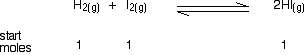Then add the "equilibrium moles" line by letting x be the number of moles of hydrogen used up. In this case, x moles of iodine will also be used up, because they react 1:1. But the hydrogen iodide is more complicated. For every mole of hydrogen that gets used up, 2 moles of HI are formed. In this case, that means that you will have an extra 2x moles of HI - a total of 1 + 2x.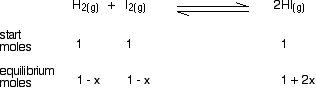Finally, convert the moles into concentrations by dividing by the volume of the mixture.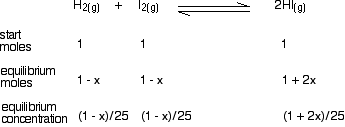Now we have got all the information we need to put into the equilibrium constant expression: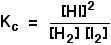Putting the values in gives: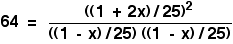When you sort this out, you will find that all the volumes cancel to leave: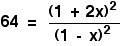Again, the right-hand side has perfect squares on both top and bottom of the expression, and so you can take the square root of both sides of the equation to get rid of all the awkward square terms. Note that the number on the left-hand side doesn't have to be an obviously recognisable perfect square like 25 or 36 or 64. I have chosen one to make the algebra look easier, but it could be any number you are given. For example, if your equilibrium constant had a value of, say, 40, then you would just take the square root of that (6.32), and use that in the rest of the calculation. Anyway, our simpler number gives this algebra:That means that at equilibrium you have got: (1 - 0.7) = 0.3 mol of both the hydrogen and the iodine. (1 + (2 x 0.7)) = 2.4 mol of hydrogen iodide. And finally . . . don't forget to check whether this is a sensible answer or not. The amount of hydrogen used up has got to lie somewhere between 0 and 1, because you are only starting from 1 mole. Our answer was 0.7, so that's perfectly reasonable. ``` ``` Go to the Section 7 Menu . . . To return to the list of learning outcomes in Section 7 Go to the CIE Main Menu . . . To return to the list of all the CIE sections Go to Chemguide Main Menu . . . This will take you to the main part of Chemguide. © Jim Clark 2019